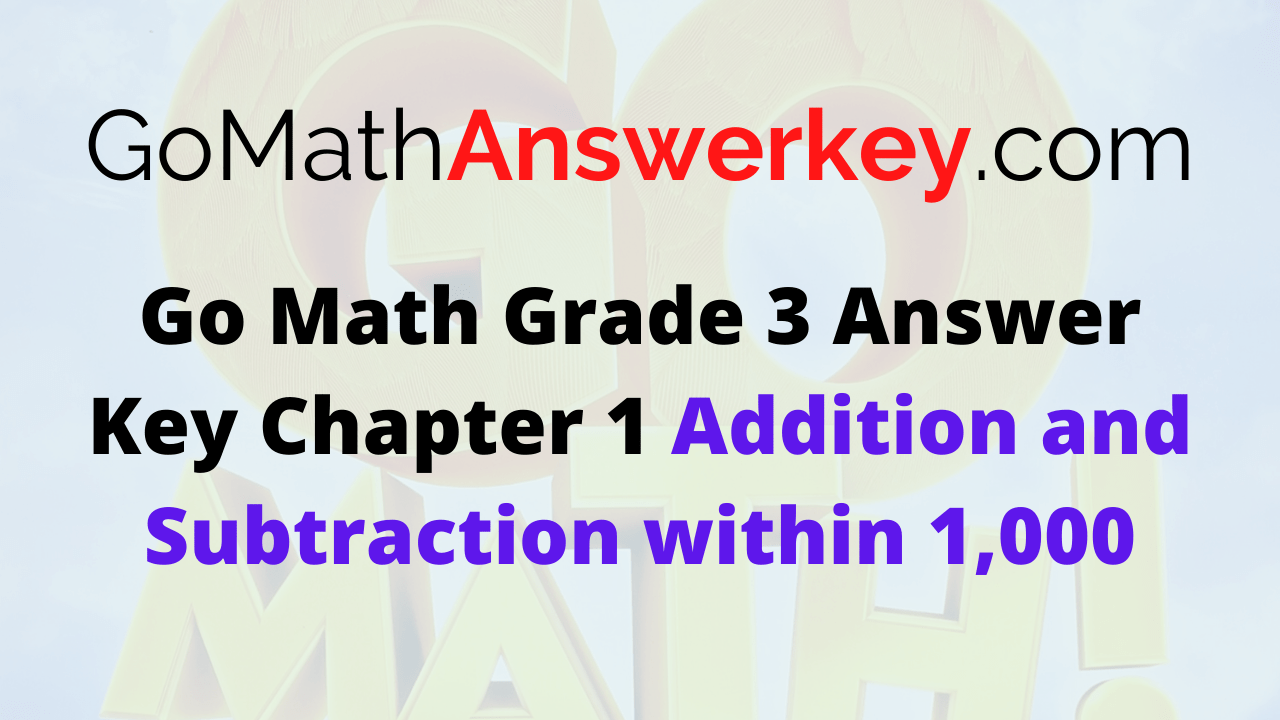Go Math Grade 3 Chapter 1 Answer Key Pdf: One has to learn the basics if he/she wants to become a master in maths. You need to practice from the beginning itself. We will help you to achieve your dreams by providing simple methods to solve the problems in an easy manner. Download Go Math Grade 3 Answer Key Chapter 1 Addition and Subtraction within 1,000 free pdf to start practicing the fundamentals of maths.

Check out the links provided in the below section to start your preparation. We have provided a brief explanation for each and every problem for your better understanding. So, make use of the links and try to score good marks in the exams. The basics not only help in the exam point of view but also in real life. There are different methods in addition and subtractions. We have solved all the methods in a simple way with easy examples.

Lesson 1: Number Patterns

Lesson 2: Round to the Nearest Ten

Lesson 3: Estimate Sums

Lesson 4: Mental Math Strategies for Addition

Lesson 5: Use Properties to Add

Lesson 6: Use the Break Apart Strategy to Add

Lesson 7: Use Place Value to Add

Mid Chapter Check Point

Lesson 8: Estimate Differences

Lesson 9: Mental Math Strategies for Subtraction

Lesson 10: Use Place Value to Subtract

Lesson 11: Combine Place Values to Subtract

Lesson 12: Problem Solving • Model Addition and Subtraction

Review/Test

### Number Patterns – Page No. 9

Find the sum. Then use the Commutative Property of Addition to write the related addition sentence.
Question 1:
9 + 2 =  11

What is the commutative property of addition?
To “commute” means to move around or travel.
According to the commutative property of addition, changing the order of the numbers we are adding, does not change the sum.
if you are adding nine and two together, the commutative property of addition says that you will get the same answer whether you are adding
9 + 2 or 2 + 9.
2 + 9 =  11

Question 2:
4 + 7 =
+      =  11

If you are adding four and seven together, the commutative property of addition says that you will get the same answer whether you are adding 4 + 7 or 7 + 4.
4 + 7 =  11
7  +  4  =  11

Question 3:
3 + 6 =
+      =  11

According to the commutative property of addition, changing the order of the numbers we are adding, does not change the sum.
There will be no change in the sum whether you add 3 + 6 or 6 + 3.
3 + 6 =  9
6  +  3  =  9

Question 4:
3 + 10 =
+      =  11

According to the commutative property of addition, changing the order of the numbers we are adding, does not change the sum.
There will be no change in the sum whether you add 3 + 10 or 10 + 3 = 13.
3 + 10 =  13
10  +  3  =  13

Question 5:
6 + 7 =
+      =  13

According to the commutative property of addition, changing the order of the numbers we are adding, does not change the sum.
There will be no change in the sum whether you add 6 + 7 or 7 + 6 = 13.
6 + 7 =  13
7  +  6  =  13

Question 6:
7 + 5 =
+      =  12

If you are adding five and seven together, the commutative property of addition says that you will get the same answer whether you are adding 7 + 5 or 5 + 7 = 12
7 + 5 =  12
5  +  7  =  12

Question 7:
8 + 9 =
+      =  17

If you are adding eight and nine together, the commutative property of addition says that you will get the same answer whether you are adding 8 + 9 or 9 + 8.
8 + 9 =  17
9  +  8  =  17

Question 8:
0 + 4 =
+      =  4

According to the commutative property of addition, changing the order of the numbers we are adding, does not change the sum.
0 + 4 =  4
4  +  0  =  4

Question 9:
9 + 6 =
+      =  15

According to the commutative property of addition, changing the order of the numbers we are adding, does not change the sum.
There will be no change in the sum whether you add 9 + 6 or 6 + 9 = 15
9 + 6 =  15
6  +  9  =  15

Is the sum even or odd? Write even or odd.

Even Numbers:

Any integer that can be divided exactly by 2 is an even number. The last digit is 0, 2, 4, 6 or 8
Example: −24, 0, 6 and 38 are all even numbers

Odd Numbers:

Any integer that cannot be divided exactly by 2 is an odd number. The last digit is 1, 3, 5, 7 or 9
Example: −3, 1, 7 and 35 are all odd numbers
Odd numbers are in between the even numbers.

Question 10:
5 + 2

The sum of two odd numbers is an odd number.
5 + 2 = 7.
∴ 7 is an odd number.

Question 11:
6 + 4

The sum of two even numbers is always an even number.
6 + 4 = 10.
∴ 10 is an even number.

Question 12:
1 + 0

The Sum of any number with zero is always the same number.
1 + 0 = 1.
∴ 1 is an odd number.

Question 13:
5 + 5

Any integer that can be divided exactly by 2 is an even number.
5 + 5 = 10.
∴ 10 is an even number.

Question 14:
3 + 8

The sum of an even and odd number is an odd number.
3 + 8 = 11.
∴ 11 is an odd number.

Question 15:
7 + 7

7 + 7 = 14.
∴ 14 is an even number.

Question 16:
Ada writes 10 + 8 = 18 on the board. Maria wants to use the Commutative Property of Addition to rewrite Ada’s addition sentence. What number sentence should Maria write?

According to the commutative property of addition, changing the order of the numbers we are adding, does not change the sum.
If you are adding ten and eight together, the commutative property of addition says that you will get the same answer whether you are adding 10 + 8 or 8 + 10.
Maria should write 8 + 10 =18.

Question 17:
Jackson says he has an odd number of model cars. He has 6 cars on one shelf and 8 cars on another shelf. Is Jackson correct? Explain.

Jackson has 6 cars on one shelf and 8 cars on another shelf, hence the total number of cars Jackson has = sum of the cars on both shelves = 6 + 8 = 14.
14 is an even number ends with ‘4’ in the last digit.
Given Statement is False, Jackson has an even number of model cars i.e. 14.

### Number Patterns Lesson Check Page No 10

Question 1
Marvella says that the sum of her addends is odd. Which of the following could be Marvella’s addition problem?

Options:
(a) 5 + 3
(b) 9 + 7
(c) 2 + 8
(d) 5 + 6

(a) 5 + 3 = 8 (Even Number)
(b) 9 + 7 = 16 (Even Number)
(c) 2 + 8 = 10 (Even Number)
(d) 5 + 6 = 11 (Odd Number)

Option (d) is Correct.

Question 2
Which number sentence shows the Commutative Property of Addition?
3 + 9 = 12

(a) 12 – 9 = 3
(b) 12 = 8 + 4
(c) 9 + 3 = 12
(d) 12 – 3 = 9

3 + 9 = 12 and 9 + 3 = 12 are commutative.
Option (c) is correct.

Spiral Review
Question 3
Amber has 2 quarters, a dime, and 3 pennies. How much money does Amber have?

(a) 53 ¢
(b) 58 ¢
(c) 63 ¢
(d) 68 ¢

Amber has
2 quarters = 2 x 25 = 50 ¢
1 dime = 10 ¢
3 pennies = 3 ¢
∴ Money does Amber have = 2 quarters + 1 dime + 3 pennies
= 50 ¢ + 10 ¢ + 3 ¢ = 63 ¢
Option (c) is correct.

Question 4
Josh estimates the height of his desk. Which is the best estimate?

(a) 1 foot
(b) 2 feet
(c) 5 feet
(d) 9 feet

So, from the given choices, 2 feet or approximately 24 inches would be the most viable answer because most standard desks have a height around 28 inches to 30 inches. 1 foot is too low, while 5 feet and 9 feet are too high. Therefore, 3 to 4 feet would be the most perfect answer but since we have a limited number of options, the nearest reasonable estimate value would be 2 feet. The original numbers from a problem does not exceed in a reasonable estimate.

Option (b) is correct.

Use the bar graph for 5–6.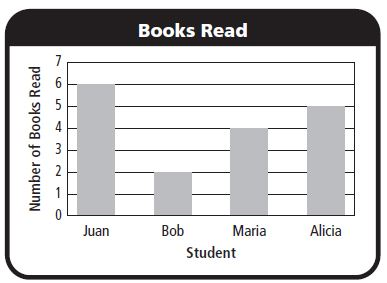Question 5

(a) Alicia
(b) Bob
(c) Juan
(d) Maria

Juan – 6
Bob – 2
Maria – 4
Alicia – 5
Option (c) is correct.

Question 6
Who read 3 more books than Bob?

(a) Alicia
(b) Juan
(c) Maria
(d) no one

Option (a) is correct.

### Lesson 2: Round to the Nearest Ten Page 15

Round to the Nearest Ten or Hundred

Locate and label 739 on the number line.
Round to the nearest hundred.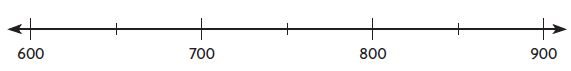Question 1

(i) 739 is between __ and __

739 is between 700 and 800.

Question 2

739 is closer to       than it is to      .

739 is closer to  700 than it is to  800.

Round to the nearest ten and hundred.

Round to the nearest ten

Rounding Numbers to the nearest 10 means finding which 10 they are nearest to. For example, 68 rounded to the nearest 10 is 70.

Rule for rounding to the nearest 10

Look at the number in the one’s place and…Work through the examples below that show rounding to the nearest 10.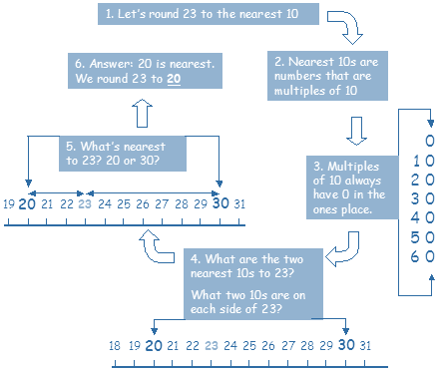Round to the nearest hundred

Rounding numbers to the nearest 100 means finding which 100 they are nearest to. For example, 680 rounded to the nearest 100 is 700.

Rule for rounding to the nearest 100

Look at the number in the tens’ place and…Work through the examples below that show rounding to the nearest 100.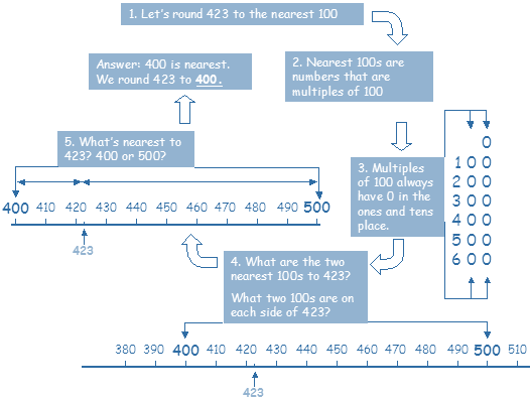Question 3
739 rounded to the nearest hundred is

Let’s round 739 to the nearest 100.
The nearest 100’s on both sides of 739 are 700 and 800.
700 is the nearest 100 to 739.
∴ 739 rounded to the nearest hundred is ‘700’

Question 4
363
Round to nearest ten:
Round to nearest hundred:

(i) Let’s round 363 to the nearest 10.

The nearest 10’s on both sides of 363 are 360 and 370.
360 is the nearest 10 to 363.
∴ 363 rounded to the nearest ten is ‘360’
Round to nearest ten: 360

(ii) Let’s round 363 to the nearest 100.

The nearest 100’s on both sides of 363 are 300 and 400.
400 is the nearest 100 to 363.
∴ 363 rounded to the nearest hundred is ‘400’
Round to nearest hundred: 400

Question 5
829
Round to nearest ten:
Round to nearest hundred:

(i) Lets round 829 to the nearest 10.
The nearest 10’s on both sides of 829 is 820 and 830.
Round to nearest ten: 830

(ii) Let’s round 829 to the nearest 100
The nearest 100’s on both sides of 829 is 800 and 900.
The number rounded to 829 nearest to 100 is 800.
Round to nearest hundred: 800

Question 6
572
Round to nearest ten:
Round to nearest hundred:

(i) Lets round 572 to the nearest 10.
The nearest 10’s on both sides of 572 is 560 and 570.
The number rounded to 572 is 570.
Round to nearest ten: 570

(ii) Let’s round 572 to the nearest 100
The nearest 100’s on both sides of 572 is 500 and 600.
The number rounded to 572 nearest to 100
Round to nearest hundred: 600

Question 7
209
Round to nearest ten:
Round to nearest hundred:

(i) Lets round 209 to the nearest 10.
The nearest 10’s on both sides of 209 is 200 and 210.
The number rounded to Round to nearest ten: 210

(ii) Let’s round 209 to the nearest 100
The nearest 100’s on both sides of 209 are 200 and 300.
The number rounded to 209 nearest to 100 is 200.
Round to nearest hundred: 200

Question 8
663
Round to nearest ten:
Round to nearest hundred:

(i) Lets round 663 to the nearest 10.
The nearest 10’s on both sides of 663 are 660 and 670.
Round to nearest ten: 660

(ii) Let’s round 663 to the nearest 100
The nearest 100’s on both sides of 663 are 600 and 700.
The number rounded to 663 nearest to 100 is 700.
Round to nearest hundred: 700

Question 9
949
Round to nearest ten:
Round to nearest hundred:

(i) Lets round 949 to the nearest 10.
The nearest 10’s on both sides of 949 is 940 and 950.
Round to nearest ten: 950

(ii) Let’s round 949 to the nearest 100
The nearest 100’s on both sides of 949 are 900 and 1000.
The number rounded to 949 nearest to 100 is 900.
Round to nearest hundred: 900

Question 10
762
Round to nearest ten:
Round to nearest hundred:

(i) Lets round 762 to the nearest 10.
The nearest 10’s on both sides of 762 is 760 and 770.
Round to nearest ten:  760

(ii) Let’s round 762 to the nearest 100
The nearest 100’s on both sides of 762 are 700 and 800.
The number rounded to 762 nearest to 100 is 800.
Round to nearest hundred:  800

Question 11
399
Round to nearest ten:
Round to nearest hundred:

(i) Lets round 399 to the nearest 10.
The nearest 10’s on both sides of 399 is 390 and 400.
Round to nearest ten: 400

(ii) Let’s round 399 to the nearest 100
The nearest 100’s on both sides of 399 are 300 and 400.
The number rounded to 399 nearest to 100 is 400
Round to nearest hundred: 400

Question 12
402
Round to nearest ten:
Round to nearest hundred:

(i) Lets round 402 to the nearest 10.
The nearest 10’s on both sides of 402 is 400 and 410.
Round to nearest ten: 400

(ii) Let’s round 402 to the nearest 100
The nearest 100’s on both sides of 402 are 400 and 500.
The number rounded to 402 nearest to 100 is 400.
Round to nearest hundred: 400

Problem Solving

Question 13
The baby elephant weighs 435 pounds. What is its weight rounded to the nearest hundred pounds?
pounds

Let’s round 435 to the nearest hundred pounds.
The nearest 100’s on both sides of 435 are 400 and 500.
400 is the nearest 100 to 435.
∴ 435 rounded to the nearest hundred pounds is ‘400’
Round to nearest hundred: 400 pounds

Question 14
Jayce sold 218 cups of lemonade at his lemonade stand. What is 218 rounded to the nearest ten?
cups

Let’s round 218 to the nearest 10.
The nearest 10’s on both sides of 218 are 210 and 220.
218 is the nearest 10 to 220.
∴ 218 rounded to the nearest ten is ‘220’
Round to nearest ten: 220

### Lesson 2: Round to the Nearest Ten Lesson Check Page No 16

Lesson Check

Question 1
One day, 758 people visited the Monkey House at the zoo. What is 758 rounded to the nearest hundred?

(a) 700
(b) 760
(c) 800
(d) 860

Let’s round 758 to the nearest hundred.
The nearest hundred on both sides is 700 and 800.
∴ 758 rounded to the nearest hundred is 800.
So, the answer is option C.

Question 2
Sami ordered 132 dresses for her store. What is 132 rounded to the nearest ten?

(a) 100
(b) 130
(c) 140
(d) 200

Let’s round 132 to the nearest 10.
The nearest 10’s on both sides of 132 are 130 and 140.
∴ 132 rounded to the nearest ten is ‘130’
132 rounded to the nearest ten: 130
Option B is the correct answer.

Spiral Review
Question 3
Which describes the number sentence?
6 + 0 = 6

(c) even + odd = odd
(d) odd + odd = odd

The Identity Property of Zero, also called the Additive Identity Property, states that if you add 0 to any number, the result will be that number. Likewise, if you subtract 0 from any number, the result will be that number.
6 + 0 = 6
∴ Option B is the correct answer.

Question 4
Which has an even sum?

(a) 7 + 4
(b) 2 + 6
(c) 5 + 4
(d) 3 + 2

The sum of even numbers is always even.
(a) 7 + 4 = 11 is odd number
(b) 2 + 6 = 8 is even number
(c) 5 + 4 = 9 is odd number
(d) 3 + 2 = 5 is odd number
So, the answer is option (b)

Question 5
What name describes this shape?(a) cone
(b) cube
(c) rectangle
(d) triangle

∴ Option D is the correct answer.

Question 6
What word describes the equal shares of the shape?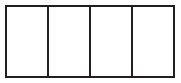(a) wholes
(b) thirds
(c) halves
(d) fourths

The rectangle is divided into 4 equal rectangles.
So, the name for the equal shapes is fourths.
∴ The answer is option D.

### Estimate Sums Page No – 21

Compatible Numbers:

Compatible numbers are the numbers that are easy to compute mentally and are close to the real numbers.

Use rounding or compatible numbers to estimate the sum.

Question 1
198 + 727 =

200 +725 = 925

Explanation:

Step 1:
First round 198 to the nearest hundred.
The number rounded to 198 nearest hundred is 200.
Write zeros for the tens and ones digit.

Step 2:
Write the number closer to 727.
The number closer to 727 is 725.

Step 3:
Now find the sum of the rounded numbers.
200 + 725 = 925

Question 2
87 + 34

Estimate:

+         =

Answer: 90 + 30 = 120

Explanation:

Step 1:
First round 87 to the nearest ten.
The number rounded to 87 nearest ten is 90.
Write zeros for the ones digit.

Step 2:
Write the number closer to 34.
The number closer to 34 is 30.

Step 3:
Now find the sum of the rounded numbers.
90 + 30 = 120

Question 3
222 + 203

Estimate:

+         =

Explanation:

Step 1:
First round 222 to the nearest hundred.
The number rounded to 222 nearest hundred is 200.
Write zeros for the tens and ones digit.

Step 2:
Write the number closer to 203.
The number closer to 203 is 200.

Step 3:
Now find the sum of the rounded numbers.
200 + 200 = 400
The estimated sum of 222 + 203 is 400.

Question 4
52 + 39

Estimate:

+         =

Answer: 50 + 40 = 90

Explanation:

Step 1:
First round 52 to the nearest ten.
The number rounded to 52 nearest ten is 50.
Write zeros for the ones digit.

Step 2:
Write the number closer to 39.
The number closer to 39 is 40.

Step 3:
Now find the sum of the rounded numbers.
50 + 40 = 90

Question 5
256 + 321

Estimate:

+         =

Answer: 250 + 325 = 575

Explanation:

Step 1:
First round 256 to the nearest ten.
The number rounded to 256 nearest ten is 250.
Write zeros for the ones digit.

Step 2:
Write the number closer to 321.
The number closer to 321 is 325.

Step 3:
Now find the sum of the rounded numbers.
250 + 325 = 575
The estimated sum of 256 + 321 is 575.

Question 6
302 + 412

Estimate:

+         =

Answer: 300 + 400 = 700

Step 1:
First round 302 to the nearest ten.
The number rounded to 302 nearest ten is 300.
Write zeros for the ones digit.

Step 2:
Write the number closer to 412.
The number closer to 412 is 400.

Step 3:
Now find the sum of the rounded numbers.
300 + 400 = 700

Question 7
519 + 124

Estimate:

+         =

Answer: 500 + 100 = 600

Explanation:

Step 1:
First round 519 to the nearest hundred.
The number rounded to 519 nearest hundred is 500.
Write zeros for the tens and ones digit.

Step 2:
Write the number closer to 124.
The number closer to 124 is 100.

Step 3:
Now find the sum of the rounded numbers.
500 + 100 = 600
The estimated sum is 600.

Question 8
790 + 112

Estimate:

+         =

Answer: 800 + 100 = 900

Explanation:

Step 1:
First round 790 to the nearest hundred.
The number rounded to 790 nearest hundred is 800.
Write zeros for the tens and ones digit.

Step 2:
Write the number closer to 112.
The number closer to 112 is 100.

Step 3:
Now find the sum of the rounded numbers.
800 + 100 = 900
The estimated sum of 790 + 112 is 900.

Question 9
547 + 326

Estimate:

+         =

Answer: 550 + 325 = 875

Explanation:

Step 1:
First round 547 to the nearest ten.
The number rounded to 547 nearest ten is 550.
Write zeros for the ones digit.

Step 2:
Write the number closer to 326.
The number closer to 326 is 325.

Step 3:
Now find the sum of the rounded numbers.
550 + 325 = 875

Question 10
325 + 458

Estimate:

+         =

Answer: 325 + 500 = 825

Explanation:

First round 458 to the nearest hundred.
The number rounded to 458 nearest hundred is 500.
Write zeros for the tens and ones digit.
You get, 325 + 500 = 825

Question 11
620 + 107

Estimate:

+         =

Answer: 600 + 100 = 700

Explanation:

The number closer to 620 is 600.
And the number closer to 107 is 100.
600 + 100 = 700
Now the estimated sum of 620 + 107 = 700

Problem Solving
Question 12
Stephanie read 72 pages on Sunday and 83 pages on Monday. About how many pages did Stephanie read during the two days?

Explanation:

Stephanie read 72 pages on Sunday and 83 pages on Monday.
The number rounded to 72 is 70 and the number rounded to 83 is 80.
To know how many pages he read in two days we need to add the number of pages he read.
70 + 80 = 150 pages.

Question 13
Matt biked 345 miles last month. This month he has biked 107 miles. Altogether, about how many miles has Matt biked last month and this month?

Explanation:

Matt biked 345 miles last month.
This month he has biked 107 miles.
The number rounded to 345 is 350.
And the number closer to 107 is 100.
Now add number of miles he rides in last month and this month.
350 + 100 = 450 miles.

### Estimate Sums Lesson Check – Page No – 22

Lesson Check
Question 1
The McBrides drove 317 miles on one day and 289 on the next day. What is the best estimate of the number of miles the McBrides drove in all during the two days?

(a) 100
(b) 400
(c) 500
(d) 600

Explanation:

The McBrides drove 317 miles on one day and 289 on the next day.
First, round 317 to the nearest hundred.
The number rounded to 317 nearest hundred is 300.
Write zeros for the tens and ones digit.
Next round 289 to the nearest hundred.
The number rounded to 289 nearest hundred is 300.
Write zeros for the tens and ones digit.
300 +300 = 600.
Option D is the correct answer.

Question 2
Ryan counted 63 birds in his backyard last week. This week, he counted 71 birds in his backyard. About how many birds did Ryan count in all?

Explanation:

Ryan counted 63 birds in his backyard last week. This week, he counted 71 birds in his backyard.
The number closer to 63 is 60.
The number closer to 71 is 70.
Now add 60 and 70 we get 130.
Therefore Ryan count about 130 birds.
So, the correct answer is option C.

Spiral Review
Question 3
What name describes this shape?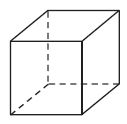(a) cone
(b) cube
(d) square

A cube is a three-dimensional solid object bounded by six square faces, facets or sides, with three meeting at each vertex. The cube is the only regular hexahedron and is one of the five Platonic solids. It has 6 faces, 12 edges, and 8 vertices.

Question 4
Which has an odd sum?

(a) 9 + 9
(b) 5 + 3
(c) 6 + 7
(d) 2 + 8

Explanation:

(a) 9 + 9 = 18 even number
(b) 5 + 3 = 8 even number
(c) 6 + 7 = 13 odd number
(d) 2 + 8 = 10 even number
So, the answer is option C.

Question 5
What is 503 rounded to the nearest hundred?

(a) 500
(b) 510
(c) 600
(d) 610

The number 503 rounded to the nearest hundred is 500.
So, the correct answer is option A.

Question 6
What is 645 rounded to the nearest ten?

(a) 600
(b) 640
(c) 650
(d) 700

645 rounded to the nearest ten is 650.
So, the correct answer is option C.

### Mental Math Strategies for Addition Page No – 27

Count by tens and ones to find the sum.

Use the number line to show your thinking.

Question 1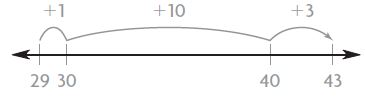Answer: 29 + 14 = 43

Question 236 + 28 =

Answer: 36 + 28 = 64

Question 345 + 26 =

Answer: 45 + 26 = 71

Question 452 + 34 =

Answer: 52 + 34 = 86

Use mental math to find the sum.

Draw or describe the strategy you use.

Question 5
52 + 19 =

Answer: 52 + 19 = 71

I Used friendly numbers.
Subtract 2 from 52.
52 – 2 = 50
19 + 2 = 21
50 + 21 = 71

Question 6
122 + 306 =

Answer: 122 + 306 = 428

I Used compatible numbers
122 = 120 + 2
306 = 300 + 6
120 + 300 = 420
2 + 6 = 8
420 + 8 = 428

Problem Solving
Question 7
Shelley spent 17 minutes washing the dishes. She spent 38 minutes cleaning her room. Explain how you can use mental math to find how long Shelley spent on the two tasks

minutes

Answer: 20 + 35 = 55 minutes

Explanation:

Shelley spent 17 minutes washing the dishes.
She spent 38 minutes cleaning her room.
First, make friendly numbers.
17 + 3 = 20
Next, subtract 3 from 38.
38 – 3 = 35
Now add both, 35 + 20 = 55
Shelly spent 55 minutes on the two tasks.

Question 8
It took Marty 42 minutes to write a book report. Then he spent 18 minutes correcting his report. Explain how you can use mental math to find how long Marty spent on his book report.

minutes

Answer: 50 + 10 = 60 minutes

Explanation:

It took Marty 42 minutes to write a book report.
Then he spent 18 minutes correcting his report.
Make a friendly number
Subtract 2 from 42
42 – 2 = 40 minutes
Now add 2 to 18 minutes
18 + 2 = 20 minutes
Now add both, 20 + 40 = 60 minutes
Therefore Marty spent 60 minutes on his book report

### Mental Math Strategies for Addition Page No – 28

Lesson Check
Question 1
Sylvia spent 36¢ for a pencil and 55¢ for a notepad. Use mental math to find how much she spent in all.

(a) 80¢
(b) 81¢
(c) 90¢
(d) 91¢

Explanation:

Sylvia spent 36¢ for a pencil and 55¢ for a notepad.
Step 1:
Make a friendly number
36¢ + 55¢ = 91¢
So, the correct answer is option D.

Question 2
Will spent 24 minutes putting together a model plane. Then he spent 48 minutes painting the model. How long did Will spend working on the model plane?

(a) 62 minutes
(b) 68 minutes
(c) 72 minutes
(d) 81 minutes

Explanation:

Will spent 24 minutes putting together a model plane. Then he spent 48 minutes painting the model.
24 + 48 = 72 minutes
Option C is the correct answer.

Spiral Review

Question 3
What name describes this shape?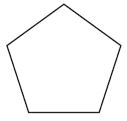(a) hexagon
(b) pentagon
(d) triangle

Explanation:

From the figure, we can observe that there are 5 sides. A pentagon is a 5-sided Polygon
So, the correct answer is option B.

Question 4
What word describes the equal shares of the shape?(a) fourths
(b) halves
(c) sixths
(d) thirds

Explanation:

The circle is divided into 4 equal parts. The name for the equal shares of circle is fourths.

Question 5
Tammy wrote an addition problem that has an odd sum. Which could be Tammy’s addition problem?

(a) 2 + 6
(b) 3 + 5
(c) 5 + 6
(d) 7 + 7

Explanation:

(a) 2 + 6 = 8 even number
(b) 3 + 5 = 8 even number
(c) 5 + 6 = 11 odd number
(d) 7 + 7 = 14 even number
11 is an odd number. So, the correct answer is option C.

Question 6
Greg counted 83 cars and 38 trucks in the mall parking lot. Which is the best estimate of the total number of cars and trucks Greg counted?

(a) 100
(b) 110
(c) 120
(d) 130

Explanation:

Greg counted 83 cars and 38 trucks in the mall parking lot.
The number closer to 83 is 80.
And the number closer to 38 is 40.
80 + 40 = 120.
So, the correct answer is option C.

### Use Properties to Add Page No 33

Use addition properties and strategies to find the sum.

Question 1Question 2
27 + 68 + 43 =

Explanation:

Step 1:
Line up the numbers by place value.
27
68
+43

Step 2:
Group the ones to make them easy to add.
Make a 10
27
68
+43

7 + 3 = 10
1 will be carried to tens place.
8 will be in the ones place.

Step 3:
Group the tens to make them easy to add.
27
68
+43
6 + 4 = 10
10 + 3 = 13

27 + 68 + 43 = 138

Question 3
42 + 36 + 18 =

Explanation:

Step 1:
Line up the numbers by place value.

42
36
+18

8 + 2 = 10
1 will be carried to the tens place
6 will be in the ones place.

Step 2:
Group the ones to make them easy to add.
Make a 10
42
36
+18

40 + 30 + 10 + 10 = 90

Step 3:
Group the tens to make them easy to add.
90 + 6 = 96

Question 4
74 + 35 + 16 + 45 =

Explanation:

Step 1:
Line up the numbers by place value.
74
35
16
+45

Step 2:
Group the ones to make them easy to add.
Make a 10
74
35
16
+45

6 + 4 = 10
5 + 5 = 10
10 + 10 = 20
2 will be carried to tens place
0 will be in the ones place.

Step 3:
Group the tens to make them easy to add.
70 + 30 + 10 + 40 +20 = 170

Question 5
41 + 26 + 149 =

Explanation:

Step 1:
Line up the numbers by place value.

149
41
+26

Step 2:
Group the ones to make them easy to add.
Make a 10

149
41
+26

9 + 1 = 10
1 will be carried to tens place.
6 will be in the ones place.

Step 3:
Group the tens to make them easy to add.
140 + 40 + 20 + 10 = 210
210 + 6 = 216

Question 6
52 + 64 + 28 + 44 =

Explanation:

Step 1:
Line up the numbers by place value.
52
64
28
+44

Step 2:
Group the ones to make them easy to add.
Make a 10

52
64
28
+44

8 + 2 = 10
4 + 4 = 8
1 will be carried to the tens place.
8 will be in the ones place.

Step 3:
Group the tens to make them easy to add.
50 + 60 + 20 + 40 + 10 = 180
180 + 8 = 188

Problem Solving
Question 7
A pet shelter has 26 dogs, 37 cats, and 14 gerbils. How many of these animals are in the pet shelter in all?

animals

Explanation:

Given that, A pet shelter has 26 dogs, 37 cats, and 14 gerbils.
Add the total number of pets
26 + 37 + 14 = 77 animals.

Question 8
The pet shelter bought 85 pounds of dog food, 50 pounds of cat food, and 15 pounds of gerbil food. How many pounds of animal food did the pet shelter buy?

pounds

Explanation:

Step 1:
Line up the numbers by place value.

85
50
+15

Step 2:
Group the ones to make them easy to add.
Make a 10

85
50
+15

5 + 5 = 10

Step 3:
Group the tens to make them easy to add.
80 + 50 + 10 + 10 = 150

### Use Properties to Add Page No 34

Lesson Check
Question 1
At summer camp there are 52 boys, 47 girls, and 18 adults. How many people are at summer camp?

(a) 97
(b) 107
(c) 117
(d) 127

Explanation:

At summer camp there are 52 boys, 47 girls, and 18 adults.
57
47
+18

52 + 47 + 18 = 117
Therefore 117 people are at summer camp.
The correct answer is option C.

Question 2
At camp, 32 children are swimming, 25 are fishing, and 28 are canoeing. How many children are swimming, fishing, or canoeing?

(a) 75
(b) 85
(c) 95
(d) 105

Explanation:

At camp, 32 children are swimming, 25 are fishing, and 28 are canoeing.
32
25
+28

Make a group of 10.

32
25
+28

8 + 2 = 10
1 will be carried to the tens place.
5 will be in the ones place.
30 + 20 + 20 + 10 = 80
80 + 5 = 85
The correct answer is option B.

Spiral Review
Question 3
Four students estimated the width of the door to their classroom. Who made the best estimate?

(a) Ted: 1 foot
(b) Hank: 3 feet
(c) Ann: 10 feet
(d) Maria: 15 feet

Question 4
Four students estimated the height of the door to their classroom. Who made the best estimate?

(a) Larry: 1 meter
(b) Garth: 2 meters
(c) Ida: 14 meters
(d) Jill: 20 meters

Question 5
Jeff’s dog weighs 76 pounds. What is the dog’s weight rounded to the nearest ten pounds?

(a) 70 pounds
(b) 80 pounds
(c) 90 pounds
(d) 100 pounds

Explanation:

Jeff’s dog weighs 76 pounds.
76 rounded to the nearest ten is 80.
The correct answer is option B.

Question 6
Ms. Kirk drove 164 miles in the morning and 219 miles in the afternoon. Which is the best estimate of the total number of miles she drove that day?

(a) 100 miles
(b) 200 miles
(c) 400 miles
(d) 500 miles

Explanation:

Ms. Kirk drove 164 miles in the morning and 219 miles in the afternoon.
The number closer to 164 is 200.
The number closer to 219 is 200.
Now add the total number of mile
200 + 200 = 400 miles.
The correct answer is option C.

### Use the Break Apart Strategy to Add Page No 39

Estimate. Then use the break apart strategy to find the sum.

Question 1Question 2
518 + 372

Estimate: 900

Sum:
518 = 500 + 10 + 8
+372 = 300 + 70 + 2
890     800 + 80 + 10

Question 3
473 + 123

Estimate: 600

Sum:
473 = 400 + 70 + 3
123 = 100 + 20 + 3
596 = 500 + 90 + 6

Question 4
208 + 569

Estimate: 800

Sum:
208 = 200 + 00 + 8
569 = 500 + 60 + 9
777 = 700 + 70 + 7

Question 5
731 + 207

Estimate: 900

Sum:
731 = 700 + 30 + 1
207 = 200 + 00 + 7
938 = 900 + 30 + 8

Question 6
495 + 254

Estimate: 800

Sum:
495 = 400 + 90 + 5
254 = 200 + 50 + 4
749 = 700 + 40 + 9

Problem Solving
Use the table for 7–8.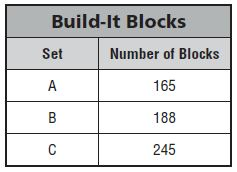Question 7
Laura is making a building using Set A and Set C. How many blocks can she use in her building?

blocks

Add set A and Set C
165 + 245 = 410 blocks

165 = 100 + 60 +5
245 = 200 + 40 + 5
410 = 300 + 100 + 10
She can use 410 blocks in her building.

Question 8
Clark is making a building using Set B and Set C. How many blocks can he use in his building?

blocks

Add Set B and Set C
188 + 245 =

188 = 100 + 80 + 8
245 = 200 + 40 + 5
433 = 300 + 120 + 13
He can use 433 blocks in his building.

### Use the Break Apart Strategy to Add Page No 40

Lesson Check
Question 1
Arthur read two books last week. One book has 216 pages. The other book has 327 pages. Altogether, how many pages are in the two books?

(a) 533
(b) 543
(c) 633
(d) 643

Explanation:

216 = 200 + 10 + 6
327 = 300 + 20 + 7
543 = 500 + 30 + 13

So, the correct answer is option B.

Question 2
One skeleton in a museum has 189 bones. Another skeleton has 232 bones. How many bones in all are in the two skeletons?

(a) 311
(b) 312
(c) 411
(d) 421

Explanation:

189 = 100 + 80 + 9
232 = 200 + 30 + 2
421 = 300 + 110 + 11
Thus the answer is option D.

Spiral Review
Question 3
Culver has 1 quarter, 3 dimes, and a penny. How much money does he have?

(a) 41¢
(b) 55¢
(c) 56¢
(d) 86¢

Explanation:

1 quarter = $0.25 1 dime =$0.10
3 dimes = $0.10 × 3 =$0.30
1 penny = $0.01 Add$0.25 + $0.30 +$0.01 = $0.56 = 56 cents Thus the correct answer is option C. Question 4 Felicia has 34 quarters, 25 dimes, and 36 pennies. How many coins does Felicia have? (a) 75 (b) 85 (c) 95 (d) 105 Answer: 95 Explanation: 1 quarter =$0.25
34 quarters = $0.25 × 34 =$8.5
25 dimes = $0.10 × 25 =$2.5
36 pennies = $0.01 × 36 = 0.36 Option C is the correct answer. Question 5 Jonas wrote 9 + 8 = 17. Which number sentence shows the Commutative Property of Addition? (a) 9 + 0 = 9 (b) 8 + 9 = 17 (c) 17 – 9 = 8 (d) 17 – 8 = 9 Answer: 8 + 9 = 17 According to the commutative property of addition, changing the order of the numbers we are adding, does not change the sum. So, the correct answer is option B. Question 6 At Kennedy School there are 37 girls and 36 boys in the third grade. How many students are in the third grade at Kennedy School? (a) 63 (b) 73 (c) 81 (d) 91 Answer: 73 Explanation: Given that, At Kennedy School there are 37 girls and 36 boys in the third grade. Add number of girls and boys = 37 + 36 = 73 Therefore the correct answer is option B. ### Use Place Value to Add Page No 45 Estimate. Then find the sum. Question 1 Estimate: 600 324 + 285 = 609 324 285 609 Question 2 519 + 347 Estimate: 500 + 300 = 800 Sum: 519 + 347 519 347 866 Question 3 323 + 151 Estimate: 323 + 151 = 325 + 150= 475 Sum: 323 151 474 Question 4 169 + 354 Estimate: 150 + 350 = 500 Sum: 169 354 523 Question 5 148 + 285 Estimate: 150 + 300 = 450 Sum: 148 + 285 = 433 148 285 433 Question 6 270 + 453 Estimate: 300 + 450 = 750 Sum: 270 + 453 = 723 270 453 723 Question 7 275 + 116 Estimate: 275 + 100 = 375 Sum: 275 116 391 Question 8 157 + 141 Estimate: 150 + 150 = 300 Sum: 157 141 298 Question 9 127 + 290 Estimate: 100 + 300 = 400 Sum: 127 290 417 Question 10 258 + 565 Estimate: 250 + 550 = 800 Sum: 258 565 823 Question 11 311 + 298 Estimate: 300 + 300 = 600 Sum: 311 298 609 Question 12 534 + 256 Estimate: 550 + 250 = 800 Sum: 534 256 790 Problem Solving Question 13 Mark has 215 baseball cards. Emily has 454 baseball cards. How many baseball cards do Mark and Emily have altogether? cards Answer: 669 baseball cards. Explanation: Given, Mark has 215 baseball cards. Emily has 454 baseball cards. Total number of baseball cards = 215 + 454 = 669 Therefore there are 669 baseball cards. Question 14 Jason has 330 pennies. Richie has 268 pennies. Rachel has 381 pennies. Which two students have more than 700 pennies combined? Answer: Jason and Rachel Explanation: Jason has 330 pennies. Richie has 268 pennies. Rachel has 381 pennies. The rounded number of 330 is 300. The number closer to 268 is 300 The number rounded to 381 is 400. You will get 700 when to combine the pennies of Jason and Rachel ### Lesson Check Page No 46 Question 1 There are 167 students in the third grade. The same number of students is in the fourth grade. How many third graders and fourth graders are there in all? (a) 224 (b) 234 (c) 324 (d) 334 Answer: 334 Explanation: Given that there are 167 students in the third grade. The same number of students is in the fourth grade. That means there are 167 students in the fourth grade. To find the total number of students in third grade and fourth grade You need to add 167 and 167 167 + 167 = 334. Thus the correct answer is option D. Question 2 Jamal read a book with 128 pages. Then he read a book with 179 pages. How many pages did Jamal read in all? (a) 397 (b) 307 (c) 297 (d) 207 Answer: 307 Explanation: Jamal read a book with 128 pages. Then he read a book with 179 pages. 128 + 179 = 307 So, the answer is option B. Spiral Review Question 3 Adam travels 248 miles on Monday. He travels 167 miles on Tuesday. Which is the best estimate for the total number of miles Adam travels? (a) 200 (b) 300 (c) 400 (d) 500 Answer: 400 Explanation: Adam travels 248 miles on Monday. He travels 167 miles on Tuesday. The number closer to 248 is 200 And the number closer to 167 is 200. 200 + 200 = 400 Thus the estimated number of miles Adam travels is 400. Question 4 Wes made$14, $62,$40, and $36 mowing lawns. How much did he make in all mowing lawns? (a)$116
(b) $152 (c)$166
(d) $188 Answer:$152

Explanation:

14
62
40
+36
152
Thus the correct answer is option B.

Question 5
There are 24 students in Mrs. Cole’s class and 19 students in Mr. Garmen’s class. How many students in all are in the two classes?

(a) 43
(b) 40
(c) 33
(d) 5

24 + 19 = 43
Thus the correct answer is option A.

Question 6
There were 475 children at the baseball game on Sunday. What is 475 rounded to the nearest ten?

(a) 400
(b) 470
(c) 480
(d) 500

Explanation:

There were 475 children at the baseball game on Sunday.
475 rounded to the nearest ten is 480.
So, the answer is option C.

### Mid Chapter Check Point – Vocabulary Page No 47

Choose the best term from the box.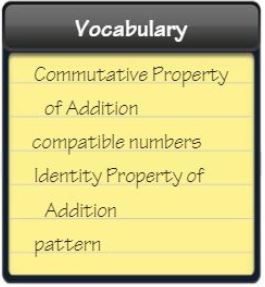Question 1
A ________ is an ordered set of numbers or objects in which the order helps you predict what comes next.

Answer: Pattern is an ordered set of numbers or objects in which the order helps you predict what comes next.

Question 2
The _________ states that when you add zero to any number, the sum is that number.

Answer: Identity property of Addition states that when you add zero to any number, the sum is that number

Concepts and Skills
Is the sum even or odd? Write even or odd.

Question 3
8 + 3

Answer: 8 + 3 = 11 is an odd number.

Question 4
9 + 7

Answer: 9 + 7 = 16 is an even number

Question 5
4 + 6

Answer: 4 + 6 = 10 is an even number

Use rounding or compatible numbers to estimate the sum.

Question 6
56+32

Estimate:

+         =

The number which is compatible to 56 is 50.
The number compatible to 32 is 25
50
25
75

50 + 25 = 75

Question 7
271+425

Estimate:

+         =

The number close to 271 is 275
425 will be the same.

275
425
700
425 + 275 = 700

Question 8
328+127

Estimate:

+         =

The number closer to 328 is 325
The number closer to 127 is 125
325 + 125 = 450

Use mental math to find the sum.

Question 9
46 + 14 =

Explanation:

Break apart the addends to make them compatible
46 = 40 + 6
14 = 10 + 4
40 + 6
10 + 4
50 + 10 = 60
46 + 14 = 60

Question 10
39 + 243 =

Explanation:

Break apart the addends to make them compatible
39 = 35 + 4
243 = 240 + 3
240 + 3
35 + 4
275 + 7

275 + 7 = 282
39 + 243 = 282

Question 11
326 + 402 =

Explanation:

Break apart the addends to make them compatible.
326 = 325 + 1
402 = 400 + 2

325 + 1
400 + 2
725 + 3 = 728
326 + 402 = 728

Estimate. Then find the sum.

Question 12
356+442
Estimate: 800
Sum: 798

356
442
798
The sum of 356 and 442 is 798
The number close to 798 is 800.
Therefore the estimated sum is 800.

Question 13
164+230
Estimate: 400
Sum: 394

230
164
394

The sum of 230 and 164 is 394
The number 394 rounded to the nearest hundred is 400.
Thus the estimated sum is 400.

Question 14
545+139
Estimate: 700
Sum: 684

545
139
684

The sum of 545 and 139 is 684.
684 rounded to the nearest hundred is 700.
So, the estimated difference is 700.

Question 15
437+184
Estimate: 600
Sum: 621

437
184
621

The sum of 437 and 184 is 621
621 rounded to the nearest hundred is 600.
The estimated sum is 600.

### Mid Chapter Check Point – Vocabulary Page No 48

Question 16
Nancy planted 77 daisies, 48 roses, and 39 tulips. About how many roses and tulips did she plant?

Explanation:

Given that, Nancy planted 77 daisies, 48 roses, and 39 tulips.
To know how many roses and tulips did she plant
We have to add a number of roses and a number of tulips.
48 and 39.
The number closer to 48 is 50.
And the number closer to 39 is 40.
So, the estimated sum is 90.

Question 17
Tomas collected 139 cans for recycling on Monday, and twice that number on Tuesday. How many cans did he collect on Tuesday?

cans

Explanation:

Tomas collected 139 cans for recycling on Monday, and twice that number on Tuesday.
Twice is nothing but double.
139 + 139 = 278
Therefore Tomas collected 278 cans on Tuesday.

Question 18
There are 294 boys and 332 girls in the Hill School. How many students are in the school?

students

Explanation:

Given,
There are 294 boys and 332 girls in the Hill School.
To find the total number of students in the students
We need to add the total number of boys and number of girls = 294 + 332
294
332
626
So, there are 626 students in the class.

Question 19
Monday’s art group made 25 paper models. Tuesday’s group made 32 paper models. Wednesday’s group made 15 paper models. How many paper models did the groups make?

paper models

Explanation:

Monday’s art group made 25 paper models.
Tuesday’s group made 32 paper models.
Wednesday’s group made 15 paper models.
25
32
15
72

### Estimate Differences Page No 53

Use rounding or compatible numbers to estimate the difference.

Question 1
40 – 13 =
40 – 10
Estimate: 30

Question 2
762 – 332

Estimate:

–          =

Estimate: 500

The number closer to 762 is 800
The number closer to 332 is 300
The difference between 800 and 300 is 500

Question 3
823 – 242

Estimate:

–          =

Estimate: 550

The number 823 rounded to the nearest hundred is 800.
The number closer to 242 is 250
800
-250
550

Question 4
98 – 49

Estimate:

–          =

Estimate: 50

The number closer to 98 is 100
The round number of 49 is 50.
100
-50
50

Question 5
287 – 162

Estimate:

–          =

Estimate: 100

282 rounded to the nearest hundred is 300
162 rounded to the nearest hundred is 200
300
-200
100

Question 6
359 – 224

Estimate:

–          =

Estimate: 125

The number closer to 359 is 350
The number closer to 224 is 225
350
-225
125

Question 7
68 – 31

Estimate:

–          =

Estimate: 40
The round number of 68 is 70
The number closer to 31 is 30
70
-30
40

Question 8
476 – 155

Estimate:

–          =

Estimate: 325

The number closer to 476 is 475
The number closer to 155 is 150
475
-150
325

Question 9
622 – 307

Estimate:

–          =

Estimate: 300

622 nearest to the hundred is 600
307 nearest to the hundred is 300
600
-300
300

Question 10
771 – 531

Estimate:

+         =

Estimate: 225

The number closer to 771 is 775
531 nearest to ten is 550
775
550
225

Question 11
299 – 61

Estimate:

+         =

Estimate: 240

The number closer to 299 is 300
The number closer to 61 is 60
300
-60
240

Problem Solving

Question 12
Ben has a collection of 812 stamps. He gives his brother 345 stamps. About how many stamps does Ben have left?

Explanation:

Ben has a collection of 812 stamps. He gives his brother 345 stamps.
812 to the nearest hundred is 800
345 to the nearest ten is 350
800
-350
450
Thus about 450 stamps are left.

Question 13
Danika is making necklaces. She has 512 silver beads and 278 blue beads. About how many more silver than blue beads does Danika have?

Explanation:

Given,
Danika is making necklaces.
The number closer to 512 is 500
278 to the nearest hundred is 300
The difference between 500 and 300 is 200.

### Lesson Check Page No 54

Question 1
Jorge has 708 baseball cards and 394 basketball cards. About how many more baseball cards than basketball cards does Jorge have?

Explanation:

Jorge has 708 baseball cards and 394 basketball cards.
The number closer to 708 is 700.
The number closer to 394 is 400
700
-400
300
So, the correct answer is option A.

Question 2
Danika is making necklaces. She has 512 silver beads and 278 blue beads. About how many more silver than blue beads does Danika have?

Explanation:

Danika is making necklaces. She has 512 silver beads and 278 blue beads.
The number closer to 512 is 500
278 to the nearest hundred is 300
The difference between 500 and 300 is 200.
So, the correct answer is option A.

Spiral Review
Question 3
A store manager ordered 402 baseball caps and 122 ski caps. Which is the best estimate of the total number of caps the manager ordered?

(a) 300
(b) 500
(c) 600
(d) 700

Explanation:

A store manager ordered 402 baseball caps and 122 ski caps.
To find the best estimate of the total number of caps the manager ordered
We have to add baseball caps and ski caps.
The number closer to 402 is 400
The number closer to 122 is 100.
400 + 100 = 500
So, the correct answer is option B.

Question 4
Autumn collected 129 seashells at the beach. What is 129 rounded to the nearest ten?

(a) 100
(b) 120
(c) 130
(d) 200

Explanation:

Autumn collected 129 seashells at the beach.
129 rounded to the nearest ten is 130
So, the correct answer is option C.

Question 5
Find the sum.

585 + 346

(a) 239
(b) 821
(c) 900
(d) 931

585
+346
931
The correct answer is option D.

Question 6
Julie made $22,$55, $38, and$25 babysitting. How much did she make in all babysitting?

(a) $102 (b)$115
(c) $140 (d)$165

Answer: $140 Explanation: Julie made$22, $55,$38, and $25 babysitting. Put all the numbers in the order 22 55 38 +25 140 So, the correct answer is option C. ### Mental Math Strategies for Subtraction Page No – 59 Use mental math to find the difference. Draw or describe the strategy you use. Question 1: 74 – 39 = 35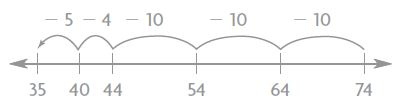Question 2 93 – 28 = Answer: 65 I use friendly numbers. Add 2 to the 93. 93 + 2 = 95 Add 2 to 28 28 + 2 = 30 95 – 30 = 65 Question 3 51 – 9 = Answer: 42 I used friendly numbers to subtract 9 from 51. Now add 1 to 9 9 + 1 = 10 Now subtract 10 from 51 51 – 10 = 41 Now add 1 to 41 41 + 1 = 42 Question 4 76 – 23 = Answer: I used friendly numbers Subtract 1 from 76 76 – 1 = 75 75 – 23 = 52 Now add 1 to 52 52 + 1 = 53. 76 – 23 = 53 Question 5 357 – 214 = Answer: I use break apart strategy. 300 – 200 = 100 50 – 10 = 40 7 – 4 = 3 100 + 40 + 3 = 143 357 – 214 = 143 Question 6 285 – 99 = Answer: I used friendly numbers. The number close to 99 is 100 285 – 100 = 185 Now add 1 to 185 185 + 1 = 186 285 – 99 = 186 Problem Solving Question 7 Ruby has 78 books. Thirty-one of the books are on shelves. The rest are still packed in boxes. How many of Ruby’s books are still in boxes? books Answer: 47 books Explanation: Ruby has 78 books. Thirty-one of the books are on shelves. The rest are still packed in boxes. To know the remaining books in the boxes. Subtract 31 from 78. 78 – 31 70 – 30 = 40 8 – 1 = 7 40 + 7 = 47 books Therefore 47 of Ruby’s books are still in boxes. Question 8 Kyle has 130 pins in his collection. He has 76 of the pins displayed on his wall. The rest are in a drawer. How many of Kyle’s pins are in a drawer? pins Answer: 54 pins Explanation: Kyle has 130 pins in his collection. He has 76 of the pins displayed on his wall. The rest are in a drawer. 130 – 76 = 54 Thus there are 54 pins in a drawer. ### Lesson Check Page No – 60 Question 1 One day, a baker made 54 fruit pies. At the end of the day, only 9 of the pies were NOT sold. How many pies were sold that day? (a) 43 (b) 45 (c) 63 (d) 65 Answer: 45 Explanation: Given, One day, a baker made 54 fruit pies. At the end of the day, only 9 of the pies were NOT sold. Number of pies sold that day = x x + 9 = 54 x = 54 – 9 = 45 x = 45 Therefore the number of pies sold that day = 45 So, the correct answer is option B. Question 2 George’s father bought a 50-pound bag of wild bird seed. At the end of two weeks, 36 pounds of seed were left in the bag. How many pounds of seed had been used? (a) 14 pounds (b) 24 pounds (c) 26 pounds (d) 86 pounds Answer: 14 pounds Explanation: George’s father bought a 50-pound bag of wild bird seed. At the end of two weeks, 36 pounds of seed were left in the bag. Number of pounds used = x x + 36 = 50 x = 50 – 36 x = 14 Therefore George’s father used 14 pounds. The correct answer is option A. Spiral Review Question 3 For a party, Shaun blew up 36 red balloons, 28 white balloons, and 24 blue balloons. How many balloons did he blow up in all? (a) 78 (b) 81 (c) 87 (d) 88 Answer: 88 Explanation: For a party, Shaun blew up 36 red balloons, 28 white balloons, and 24 blue balloons. Total number of balloons = 36 + 28 + 24 36 28 +24 88 So, the answer is option D. Question 4 Tiffany has read 115 pages of her book. She has 152 pages left to read. How many pages are in the book? (a) 37 (b) 267 (c) 277 (d) 367 Answer: 267 Explanation:; Tiffany has read 115 pages of her book. She has 152 pages left to read. Total number of pages = 152 + 115 152 +115 267 Question 5 The flower shop had 568 flowers on Monday. By Tuesday, the shop had 159 flowers left. About how many flowers had been sold? (a) about 200 (b) about 300 (c) about 400 (d) about 500 Answer: about 400 Explanation: The flower shop had 568 flowers on Monday. By Tuesday, the shop had 159 flowers left. The number closer to 568 is 600. The number closer to 159 is 200 Subtract 200 from 600. 600 – 200 = 400 The correct answer is option C. Question 6 There are 383 books in one section of the school library. Of the books, 165 are fiction books. Which is the best estimate of the number of books in that section that are NOT fiction? (a) about 200 (b) about 300 (c) about 400 (d) about 500 Answer: about 200 Explanation: There are 383 books in one section of the school library. Of the books, 165 are fiction books. 383 to the nearest hundred is 400. 165 to the nearest hundred is 200 400 – 200 = 200 So, the correct answer is option A. ### Use Place Value to Subtract Page No 65 Estimate. Then find the difference. Question 1 Estimate: 500 585 – 119 Subtract 119 from 585 585 119 466 585 – 119 = 466 Question 2 738 – 227 Estimate: 500 Difference: 511 Subtract 227 from 738 738 227 511 The estimated difference of 511 is 500. 738 – 227 = 511 Question 3 651 – 376 Estimate: 300 Difference: 275 Subtract 376 from 651 651 376 275 651 – 376 = 275 The estimated difference is 300 Question 4 815 – 281 Estimate: 500 Difference: 534 Subtract 281 from 815 815 281 534 815 – 281 = 534 The estimated difference is 500 Question 5 487 – 290 Estimate: 200 Difference: 197 487 290 197 487 – 290 = 197 The estimated difference is 200. Question 6 936 – 329 Estimate: 600 Difference: 607 936 329 607 936 – 329 = 607 The estimated difference is 600. Question 7 270 – 128 Estimate: 140 Difference: 142 Subtract 128 from 270 270 128 142 270 – 128 = 142 The estimated difference is 140. Question 8 364 – 177 Estimate: 200 Difference: 187 Subtract 177 from 364 364 177 187 364 – 177 = 187 The estimated difference is 200. Question 9 627 – 253 Estimate: 400 Difference: 374 Subtract 253 from 627 627 253 374 627 – 253 = 374 The estimated difference is 374 Question 10 862 – 419 Estimate: 450 Difference: 443 Subtract 419 from 862 862 419 443 862 – 419 = 443 The estimated difference is 450. Question 11 726 – 148 Estimate: 550 Difference: 578 Subtract 148 from 726 726 148 578 726 – 148 = 578 The estimated difference is 550. Question 12 543 – 358 Estimate: 200 Difference: 185 Subtract 358 from 543 543 358 185 543 – 358 = 185 The estimated difference is 200. Problem Solving Question 13 Mrs. Cohen has 427 buttons. She uses 195 buttons to make puppets. How many buttons does Mrs. Cohen have left? buttons Answer: 232 buttons Explanation: Mrs. Cohen has 427 buttons. She uses 195 buttons to make puppets. To find how many buttons left, we have to subtract number of buttons she used to make puppets from the total number of buttons. 427 – 195 = 232 Therefore 232 buttons are left. Question 14 There were 625 ears of corn and 247 tomatoes sold at a farm stand. How many more ears of corn were sold than tomatoes? more ears Answer: 378 more ears of corn Explanation: There were 625 ears of corn and 247 tomatoes sold at a farm stand. To know number of ears of corn were sold than tomatoes we have to subtract 247 from 625 625 -247 378 Thus 378 more ears of corn were sold than tomatoes. ### Use Place Value to Subtract Page No 66 Question 1 On Saturday, 453 people go to a school play. On Sunday, 294 people go to the play. How many more people go to the play on Saturday? (a) 159 (b) 169 (c) 259 (d) 747 Answer: 159 Explanation: On Saturday, 453 people go to a school play. On Sunday, 294 people go to the play. To find how many more people go to the play on Saturday We need to subtract number of people go to the play on Sunday from the number of people go to the play on Saturday = 453 – 294 = 159 159 more people go to the play on Saturday. Thus the correct answer is option A. Question 2 Corey has 510 marbles. He fills one jar with 165 marbles. How many of Corey’s marbles are NOT in the jar? (a) 675 (b) 455 (c) 350 (d) 345 Answer: 345 Explanation: Corey has 510 marbles. He fills one jar with 165 marbles. Let the number of Corey’s marbles are NOT in the jar be x x + 165 = 510 x = 510 – 165 x = 345 Therefore 345 marbles are NOT in the jar. The correct answer is option D. Spiral Review Question 3 Pattie brought 64 peppers to sell at the farmers’ market. There were 12 peppers left at the end of the day. How many peppers did Pattie sell? (a) 50 (b) 52 (c) 62 (d) 78 Answer: 52 Explanation: Pattie brought 64 peppers to sell at the farmers’ market. There were 12 peppers left at the end of the day. To find number of peppers did Pattie sell Subtract 12 from 64 64 – 12 = 52 The correct answer is option B. Question 4 An airplane flies 617 miles in the morning. Then it flies 385 miles in the afternoon. About how many more miles does the airplane fly in the morning? (a) about 100 miles (b) about 200 miles (c) about 300 miles (d) about 900 miles Answer: about 200 miles Explanation: An airplane flies 617 miles in the morning. Then it flies 385 miles in the afternoon. Here we have to use the concept of estimated difference. The number closer to 617 is 600 The number closer to 385 is 400 600 – 400 = 200 About 200 miles airplane fly in the morning. So, the correct answer is option B. Question 5 What is the unknown number? (■ + 4) + 59 = 70 (a) 4 (b) 6 (c) 7 (d) 8 Answer: 7 Explanation: Let ■ be the unknown number (■ + 4) + 59 = 70 (■ + 4) = 70 – 59 (■ + 4) = 11 (■ = 11 – 4 ■ = 7 Thus the correct answer is option C. Question 6 Dexter has 128 shells. He needs 283 more shells for his art project. How many shells will Dexter use for his art project? (a) 155 (b) 165 (c) 401 (d) 411 Answer: 411 Explanation: Dexter has 128 shells. He needs 283 more shells for his art project. To know the total number of shells that Dexter used for his art project you need to add 128 and 283 283 + 128 = 411 So, the correct answer is option D. ### Combine Place Values to Subtract Page No – 71 Estimate. Then find the difference. Question 1 Estimate: 200 476 – 269 476 -269 207 The estimated difference is 200. Question 2 615 – 342 Estimate: 300 Difference: 273 615 -342 273 The difference between 615 and 342 is 273 The estimated difference is 300. Question 3 508 – 113 Estimate: 400 Difference: 395 508 -113 395 The difference between 508 and 113 is 395 The estimated difference is 400 Question 4 716 – 229 Estimate: 500 Difference: 487 716 229 487 The number closer to 487 is 500. The difference is 487. Question 5 700 – 326 Estimate: 400 Difference: 374 700 326 374 The number closer to 374 is 400. The difference is 374. Question 6 325 – 179 Estimate: 100 Difference: 146 325 179 146 The number closer to 146 is 100 The difference is 146. Question 7 935 – 813 Estimate: 100 Difference: 122 935 813 122 The number closer to 122 is 100. The difference is 122. Question 8 358 – 292 Estimate: 50 Difference: 66 358 292 66 The number closer to 66 is 50. The difference is 66. Question 9 826 – 617 Estimate: 200 Difference: 209 826 617 209 The number closer to 209 is 200. The difference is 209. Question 10 900 – 158 Estimate: 750 Difference: 742 900 158 742 The number closer to 742 is 750. The difference is 742 Question 11 607 – 568 Estimate: 40 Difference: 39 607 568 39 The number closer to 39 is 40. The difference is 40. Question 12 973 – 869 Estimate: 100 Difference: 104 973 869 104 The number closer to 104 is 100. The difference is 104. Problem Solving Question 13 Bev scored 540 points. This was 158 points more than Ike scored. How many points did Ike score? points Answer: 382 points Explanation: Bev scored 540 points. This was 158 points more than Ike scored. Let the number of points Ike scored = x x + 158 = 540 x = 540 – 158 x = 382 Therefore the points that Ike scored is 382. Question 14 A youth group earned$285 washing cars. The group’s expenses were $79. How much profit did the group make washing cars?$       profit

Answer: $206 Explanation: A youth group earned$285 washing cars.
The group’s expenses were $79. To find how much profit did the group make washing cars. Subtract 79 from 285 285 – 79 285 -79 206 The group makes$206 profit by washing cars.

### Lesson 11: Combine Place Values to Subtract Page No 72

Question 1
A television program lasts for 120 minutes. Of that time, 36 minutes are taken up by commercials. What is the length of the actual program without the commercials?

(a) 84 minutes
(b) 94 minutes
(c) 104 minutes
(d) 156 minutes

Explanation:

A television program lasts for 120 minutes.
Of that time, 36 minutes are taken up by commercials.
To find the length of the actual program without the commercials
Subtract 36 minutes from 120 minutes
120
-36
84
Thus the length of the actual program without the commercials is 84 minutes.
The correct answer is option A.

Question 2
Syd spent 215 minutes at the library. Of that time, he spent 120 minutes on the computer. How much of his time at the library did Sid NOT spend on the computer?

(a) 85 minutes
(b) 95 minutes
(c) 105 minutes
(d) 335 minutes

Explanation:

Syd spent 215 minutes at the library.
Of that time, he spent 120 minutes on the computer.
To find How much of his time at the library did Sid NOT spend on the computer
We have to subtract the time he spent on the computer from the total time he spent at the library.
i.e., 215 – 120 = 95 minutes
So, the correct answer is option B.

Spiral Review
Question 3
Xavier’s older brother has 568 songs on his music player. To the nearest hundred, about how many songs are on the music player?

(a) 500
(b) 600
(c) 700
(d) 800

Explanation:

Xavier’s older brother has 568 songs on his music player.
568 to the nearest hundred is 600.
Thus the correct answer is option B.

Question 4
The students traveled to the zoo in 3 buses. One bus had 47 students. The second bus had 38 students. The third bus had 43 students. How many students in all were on the three buses?

(a) 108
(b) 118
(c) 128
(d) 138

Explanation:

The students traveled to the zoo in 3 buses.
The second bus had 38 students.
The third bus had 43 students.
Total number of students in all three buses = x
x = 47 + 38 + 43
x = 128 students.
So, the correct answer is option C.

Question 5
Callie has 83 postcards in her collection. Of the postcards, 24 are from Canada. The rest of the postcards are from the United States. How many of the postcards are from the United States?

(a) 58
(b) 59
(c) 61
(d) 69

Explanation:

Callie has 83 postcards in her collection.
Of the postcards, 24 are from Canada.
The rest of the postcards are from the United States.
Subtract 24 from 83 we get the number of postcards is from the United States.
83 – 24 = 59
So, the correct answer is option B.

Question 6
There were 475 seats set up for the school play. At one performance, 189 of the seats were empty. How many seats were filled at that performance?

(a) 286
(b) 296
(c) 314
(d) 396

Explanation:

There were 475 seats set up for the school play.
At one performance, 189 of the seats were empty.
Let the Number of seats were filled at that performance = x
x + 189 = 475
x = 475 – 189
x = 286
Thus the correct answer is option A.

### Problem Solving • Model Addition and Subtraction Page No – 77

Use the bar model to solve the problem.

Question 1
Elena went bowling. Elena’s score in the first game was 127. She scored 16 more points in the second game than in the first game. What was her total score?Question 2
Mike’s Music sold 287 CDs on the first day of a 2-day sale. The store sold 96 more CDs on the second day than on the first day. How many CDs in all were sold during the 2-day sale?CDs

Explanation:

Mike’s Music sold 287 CDs on the first day of a 2-day sale.
The store sold 96 more CDs on the second day than on the first day.
The means Mike’s music sold CDs on the second day = 287 + 96 = 383.
★ = 283 CDs
Total CDs were sold during the 2-day sale = 383 + 287
♦ = 383 + 287 = 670 CDs

### Lesson Check Page No – 78

Question 1
Ms. Hinely picked 46 tomatoes from her garden on Friday. On Saturday, she picked 17 tomatoes. How many tomatoes did she pick in all?

(a) 109
(b) 63
(c) 53
(d) 29

Explanation:

Ms. Hinely picked 46 tomatoes from her garden on Friday.
On Saturday, she picked 17 tomatoes.
First, find how many tomatoes did she pick in all.
46 + 17 = ★
★ = 63
So, the correct answer is option B.

Question 2
Rosa read 57 pages of a book in the morning. She read 13 fewer pages in the afternoon. How many pages did Rosa read in the afternoon?

(a) 44
(b) 60
(c) 70
(d) 83

Explanation:

Rosa read 57 pages of a book in the morning.
She read 13 fewer pages in the afternoon.
57 – 13 = ♦
♦ = 57 – 13
♦ = 44
Thus the correct answer is option A.

Spiral Review
Question 3
Mike has 57 action figures. Alex has 186 action figures. Which is the best estimate of the number of action figures Mike and Alex have altogether?

(a) 150
(b) 250
(c) 350
(d) 400

Explanation:

Mike has 57 action figures.
Alex has 186 action figures.
186 – 57 = ★
★ = 186 – 57
★ = 129
Now Add Mike and Alex action figures
♦ = 186 + 57 = 243
The estimated figure of 243 is 250.
Thus the correct answer is option B.

Question 4
There are 500 sheets of paper in the pack Hannah bought. She has used 137 sheets already. How many sheets of paper does Hannah have left?

(a) 363
(b) 463
(c) 400
(d) 637

Explanation:

There are 500 sheets of paper in the pack Hannah bought. She has used 137 sheets already.
To find how many sheets of paper does Hannah have left
We have to subtract the number of sheets used from the total number of sheets.
500 – 137 = ★
★ = 500 – 137
★ = 363
Therefore 343 sheets are left.
The correct answer is option A.

Question 5
There were 378 visitors to the science museum on Friday. There were 409 visitors on Saturday. How many more people visited the museum on Saturday?

(a) 25
(b) 31
(c) 171
(d) 787

Explanation:

There were 378 visitors to the science museum on Friday.
There were 409 visitors on Saturday.
To find how many more people visited the museum on Saturday.
Subtract the number of visitors on Friday from the number of visitors on Saturday.
409 – 378 = 31
31 people visited more the museum on Saturday.
So the correct answer is option B.

Question 6
Ravi scores 247 points in a video game. How many more points does he need to score a total of 650?

(a) 897
(b) 430
(c) 417
(d) 403

Explanation:

Ravi scores 247 points in a video game.
Let x be the points he needs to score a total of 650
x + 247 = 650
x = 650 – 247
x = 403
Thus he needs 403 points to make a score of 650.
The correct answer is option D.

### Review/Test – Page No 79

Question 1

For numbers 1a–1d, choose Yes or No to tell whether the sum is even.

a. 5 + 8

(a) yes
(b) no

Explanation:

5 + 8 = 13 is an odd number.

Question 1
b. 9 + 3

(a) yes
(b) no

Explanation:

9 + 3 = 12 is an even number.

Question 1
c. 6 + 7

(a) yes
(b) no

Explanation:

6 + 7 = 13 is an odd number.

Question 1
d. 9 + 5

(a) yes
(b) no

Explanation:

9 + 5 = 14 is an even number.

Question 2
Select the number sentences that show the Commutative Property of Addition. Mark all that apply.

(a) 14 + 8 = 22
(b) 8 + 14 = 14 + 8
(c) 8 + (13 + 1) = (8 + 13) + 1
(d) (5 + 9) + 8 = (9 + 5) + 8

Answer: 8 + 14 = 14 + 8

Explanation:

According to the commutative property of addition, changing the order of the numbers we are adding, does not change the sum.
So, the answer is option B.

Question 3
Select the numbers that round to 300 when rounded to the nearest hundred. Mark all that apply.

(a) 238
(b) 250
(c) 283
(d) 342
(e) 359

Explanation:
283 rounded to the nearest hundred is 300.
So, the correct answer is option C.

Question 4
There are 486 books in the classroom library. Complete the chart to show 486 rounded to the nearest 10.Hundreds Tens Ones 400 90 0

486 rounded to the nearest ten is 490.

### Review/Test – Page No – 80

Question 5
Write each number sentence in the box below the better estimate of the sum.

393+225=■ 481+215=■

352+328=■ 309+335=■600 700 393+225 = 618 The estimated sum is 600.309+335= 644 The estimated sum is 600. 481+215= 696 The estimated sum is 700.352+328= 680 The estimated sum is 700.

Explanation:

393+225=■
■ = 618
The number closer to 618 is 600

481+215=■
■ = 696
The number closer to 696 is 700

352+328=■
■ = 680
The number closer to 680 is 700.

309+335=■
■ = 644
The number closer to 644 is 600.

Question 6
Diana sold 336 muffins at the bake sale. Bob sold 287 muffins. Bob estimates that he sold 50 fewer muffins than Diana. How did he estimate? Explain.

Diana sold 336 muffins at the bake sale.
Bob sold 287 muffins.
Bob estimates that he sold 50 fewer muffins than Diana.
To know whether his estimation is right or wrong we have to subtract muffins that Bob sold from muffins that Diana sold
336 – 287 = 49
The number closer to 49 is 50.
So, Bob’s estimation is correct.

Question 7
The table shows how many books each class read.For numbers 7a–7d, select True or False for each statement.

a. Ms. Martin’s class read about 100 more books than Mr. Lopez’s class.

(i) True
(ii) False

Explanation:

Number of books that Mr. Lopez’s class read = 273
Number of books that Ms. Martin’s class read = 402
402
– 273
129
So, the statement Ms. Martin’s class read about 100 more books than Mr. Lopez’s class is true.

Question 7
b. The 3 classes read over 900 books altogether.

(i) True
(ii) False

Explanation:

Number of books that Mr. Lopez’s class read = 273
Number of books that Ms. Martin’s class read = 402
Number of books that Mrs. Wang read = 247
273
402
274
949
Therefore the statement the 3 classes read over 900 books altogether is true.

Question 7
c. Mrs. Wang’s class read about 50 fewer books than Mr. Lopez’s class.

(i) True
(ii) False

Explanation:

Number of books that Mrs. Wang read = 247
Number of books that Mr. Lopez’s class read = 273
273
– 247
26
Thus the statement Mrs. Wang’s class read about 50 fewer books than Mr. Lopez’s class is false.

Question 7

(i) True
(ii) False

Explanation:

Number of books that Ms. Martin’s class read = 402
Number of books that Mrs. Wang read = 247
402
247
649
Therefore the statement Ms. Martin’s and Mrs. Wang’s class read about 700 books is false.

### Review/Test – Page No – 81

Question 8
Janna buys 2 bags of dog food for her dogs. One bag weighs 37 pounds. The other bag weighs 15 pounds. How many pounds do both bags weigh? Explain how you solved the problem.

pounds

Explanation:

Janna buys 2 bags of dog food for her dogs. One bag weighs 37 pounds. The other bag weighs 15 pounds.
I used friendly numbers

37 = 35 + 2
15 = 15 + 0
52 =  50 + 2
The weight of 2 bags is 52 pounds.

Question 9
Choose the property that makes the statement true.

The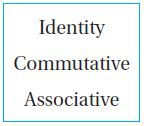Property of addition states that you can group addends in different ways and get the same sum.

Answer: The Associative Property of addition states that you can group addends in different ways and get the same sum.

Use the table for 10–12.Question 10
The table shows the number of sweaters sold online in three months. How many sweaters were sold in January and February?

sweaters

Explanation:

The number of sweets sold in the month of January = 402
The number of sweets sold in the month of February = 298
First, make the friendly numbers to make the addition easy.|
Subtract 2 from 402 = 402 – 2 = 400
Next add 2 to 298 = 298 + 2 = 300
400 + 300 = 700
Therefore 700 sweaters were sold in January and February.

Question 11
How many more sweaters were sold in January than March?

sweaters

Explanation:

The number of sweets sold in the month of January = 402
The number of sweets sold in the month of March = 171
To find how many more sweaters were sold in January than March, we have subtracted the number of sweaters sold in the march from January
402 – 171 = 231
231 more sweaters were sold in January than March.

Question 12

How many more sweaters were sold in February and March than in January?

sweaters

Explanation:

The number of sweets sold in the month of January = 402
The number of sweets sold in the month of February = 298
The number of sweets sold in the month of March = 171
Total number of sweaters sold in February and March = 298 + 171 = 469
Now subtract 402 from 469
469 – 402 = 67 sweaters
67 more sweaters were sold in February and March than in January.

### Review/Test – Page No – 82

Question 13
Help Dana find the sum.

346 + 421 + 152
For numbers 13a–13d, select Yes or No to tell Dana when to regroup.

a. Regroup the ones.

(a) yes
(b) no

Question 13

(a) yes
(b) no

Question 13
c. Regroup the tens.

(a) yes
(b) no

Question 13

(a) yes
(b) no

Question 14
Alexandra has 78 emails in her inbox. She deletes 47 emails. How many emails are left in her inbox? Draw jumps and label the number line to show your thinking.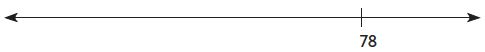emails

Explanation:

Alexandra has 78 emails in her inbox.
She deletes 47 emails.
Let x be the number of emails left in her inbox
x + 47 = 78
x = 78 – 47
x = 31
Therefore, 31 emails are left in her inbox.

Question 15
Daniel has 402 pieces in a building set. He uses 186 pieces to build a house. How many pieces does he have left? Show your work.

pieces

Explanation:

Daniel has 402 pieces in a building set.
He uses 186 pieces to build a house.
x be the number of pieces he had left
x + 186 = 402
x = 402 – 186
x = 216 pieces
Thus he left 216 pieces to build a house

### Review/Test – Page No – 83

Question 16
Luke solves this problem. He says the difference is 214. Explain the mistake Luke made. What is the correct difference?

352−148 =

Explanation:

Make friendly numbers to make the subtraction easy.
First subtract 2 from 352 = 350
350
148
202
1 will be borrowed from tens place. So 0 becomes 10.
10 – 8 = 2
4 – 4 = 0
300 – 100 = 200
200 + 2 = 202
Now add 2 to 202 you get 204.

Question 17
Sunnyday Elementary School is having its annual Read-a-thon. The third graders have read 573 books so far. Their goal is to read more than 900 books. What is the least number of books they need to read to reach their goal? Explain.

books

Explanation:

Sunnyday Elementary School is having its annual Read-a-thon.
The third graders have read 573 books so far. Their goal is to read more than 900 books.
Let the 3rd graders have to read the total number of books = x
x + 573 = 900
x = 900 – 573
x = 327
Thus the least number of books they need to read to reach their goal is 327 books.

Question 18
There are 318 fiction books in the class library. The number of nonfiction books is 47 less than the number of fiction books.

Part A

About how many nonfiction books are there in the class library? Explain.

Explanation:

Given that,
There are 318 fiction books in the class library.
The number of nonfiction books is 47 less than the number of fiction books.
Number of non fictions books = x
x + 47 = 318
x = 318 – 47
x = 271
The number closer to 271 is 270.
So, there are about 270 nonfiction books.

Question 18
Part B

How many fiction and nonfiction books are there in the class library altogether? Show your work.

total books

Explanation:

Number of fiction books = 318
Number of nonfiction books = 271
To find the total number of books we need to add both fiction and nonfiction books
= 318 + 271 = 589
There are 589 books in the class library.

### Review/Test – Page No – 84

Question 19
Alia used 67 + 38 = 105 to check her subtraction. Which math problem could she be checking? Mark all that apply.

67−38=■
105−67=■
105+38=■
105−38=■

She can use option B and Option D to check her subtraction.

Question 20
Alex and Erika collect shells. The tables show the kinds of shells they collected.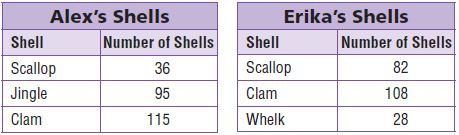Part A

Who collected more shells? How many did she collect? About how many more is that? Explain how you solved the problem.

Alxe’s Shells:
Number of Scallop = 36
Number of Jingle shells = 95
Number of Clam = 115
Now add all the three shells = 36 + 95 + 115 = 246 shells

Erika’s shells:

Number of Scallop = 82
Number of Whelk shells = 28
Number of Clam = 108
Now add all the three shells = 82 + 28 + 108 = 218 shells

Question 20
Part B

Alex and Erika have the greatest number of what kind of shell? How many shells of that kind do they have? Show your work.